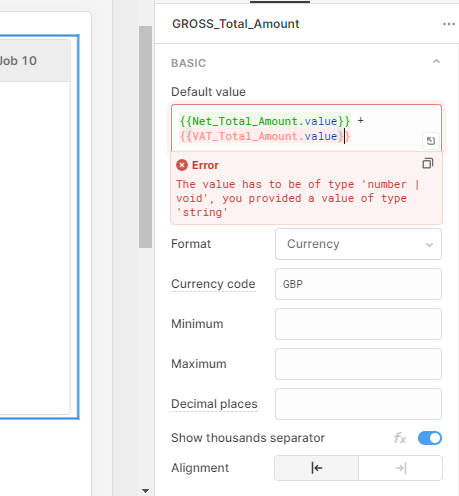Hi,

built a CRUD system so moving onto what i thought would be easy and i just feel im missing something!

I have 3 fields. all numberinputi am trying to sum the input in VAT and Net into the Gross but keep getting this errorAny help will be appreciated.

Thanks

Mike

Found the solutionyou have to create Javascript transformer with the formula you would like to use i.e.

return {{Net_Total_Amount.value}} + {{VAT_Total_Amount.value}}

then put the transport code i.e {{ Calculate_Total_Gross.value }} into the default value of the numerical input

Hey @MewWales nice that you were able to find a solution!

You can also try using `{{Net_Total_Amount.value + VAT_Total_Amount.value}}` in the default value field.

Curly braces allow you to write entire JS expressions using the values of your components, otherwise, fields tend to interpret what you write as a string.

1 Like